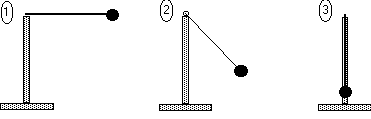Teaching Physics with the Physics Suite Edward F. Redish

Swinging a

A pendulum consisting of a massive ball on a light but nearly rigid rod is shown at three successive times in the pictures below. The maximum angle of displacement of the pendulum from its equilibrium point is 90o.1. If the length of the rod is R and the mass of the ball is m, find an expression that will give the speed of the ball at the point shown in picture 3 when numbers are put in.
2. If the ball in the third picture is moving to the left at the instant of the snapshot, in what direction does its acceleration point at this instant in time?
3. In what direction does the acceleration of the ball in the first picture point?

Note to the instructor: This is difficult for many students, even physics grad students. They tend to read the motion at the bottom of the pendulum's swing as straight line rather than circular motion. To solve it effectively, students need to have a good procedural understanding of how to construct a vector acceleration as proportional to a change in the velocity vector.

Not finding what you wanted? Check the Site Map for more information.